# Modern DACs and DAC Buffers Improve System Performance, Simplify Design

At the heart of many control systems, digital-to-analog converters (DACs) play a key role in determining system performance and accuracy. This article looks at two new precision 16-bit DACs and shows some ideas for buffering the outputs of high-speed complementary current-output DACs that can rival transformer performance.

### On-Chip system Reference Improves Accuracy

Electronic systems operating in harsh environments may have to endure large temperature extremes while maintaining their accuracy and stability. Such systems often require multiple digital-to-analog converters with resolutions up to 16 bits. The accuracy of the DAC output voltage ultimately depends on the accuracy of its reference voltage. Usually, for this level of performance over temperature, a separate precision reference would be used. Now, however, a new precision quad DAC family includes a low-drift voltage reference with performance good enough to serve as a system reference.

The voltage reference should maintain a constant voltage independent of load, power supply variation, and temperature. Unfortunately, high-performance standalone voltage references are often large, costly, and power hungry—and on-chip references often have poor performance.

Until now, faced with the choice of pairing an accurate DAC with an external reference or suffering with a low-performance on-chip reference, system designers were often forced to choose the DAC, plus external reference. They couldn’t rely on the performance of the on-chip reference, as its large variation over temperature and frequent lack of a maximum TC specification didn’t allow its use as a system reference.

The AD5686R alters this paradigm. This quad, voltage-output, 16-bit nanoDAC+® digital-to-analog converter provides an on-chip 2.5-V reference with 5 ppm/°C maximum (2 ppm/°C typical) temperature drift. This level of performance matches that of standalone alternatives, allowing the on-chip reference to replace the system reference to save space, cost, and power. The 5-ppm/°C maximum drift specification allows precise error budget calculations. The performance of the AD5686R’s on-chip reference is shown in Figure 1 and Figure 2. Figure 1 is a scatter plot of temperature coefficients for several hundred DACs comprising five separate sample lots. Figure 2 shows actual output voltage for nine devices over the –40°C to +105°C temperature range.Figure 1. Temperature coefficient of several hundred AD5686R DACs.Figure 2. Reference voltage vs. temperature for nine typical AD5686R DACs.

Method: For voltage references, temperature coefficient (TC) is usually determined using the box method, which evaluates the maximum voltage change over the specified temperature range. TC, expressed in ppm/°C, is calculated as follows

where

VREFmax is the maximum reference output measured over the temperature range.
VREFmin is the minimum reference output measured over the temperature range.
VREFnom is the nominal reference output voltage.
TempRange is the specified temperature range (°C).

The low temperature coefficient is achieved by heating the part in production and trimming the output voltage to compensate for curvature over temperature. Tight matching ensures that resistor differences don’t impact the reference’s performance and also allows the DAC to achieve an impressive ±2-LSB integral nonlinearity (INL) specification.

### About the AD5686R/AD5685R/AD5684R

The AD5686R/AD5685R/AD5684R , members of the nanoDAC+ family, are quad, low-power, 16-/14-/12-bit DACs with buffered voltage outputs. The nanoDAC+ family was developed to meet the growing need for digital-to-analog converters that provide precision, ease of operation, and small package size. These new devices include an on-chip 2.5-V, 2-ppm/°C reference, which is enabled by default. A gain select pin sets the full-scale output to 2.5 V (GAIN = 1) or 5 V (GAIN = 2). Operating from a single 2.7-V to 5.5-V supply, the devices specify 0.1% maximum gain error, 2-mV maximum offset error, and guaranteed monotonicity. Available in LFCSP (3-mm × 3-mm) and TSSOP packages, their 4-kV ESD rating highlights them as extremely robust. The DAC input is programmed via a 1.8-V SPI-compatible interface. A power-on reset circuit ensures that the DAC outputs power up to 0 V and remain there until a valid write takes place. A reset pin allows asynchronous reset. The reference out pin allows the on-chip reference to be used externally as a system reference. A daisy-chain feature enables systems with higher channel counts. External-reference versions, the AD5686/AD5685/AD5684, allow a single reference to be shared among all channels at lowest cost. A portion of a multichannel system using these DACs appears in Figure 3.Figure 3. Portion of a system block diagram showing AD5686R and AD5686 controlled by Blackfin DSP.

### Voltage-Switching, 16-Bit DACs Provide Low Noise, Fast Settling, Improved Linearity

Resistance-ladder multiplying DACs, based on the game-changing 10-bit CMOS AD7520—introduced nearly 40 years ago—were, at first, used with inverting op amps, with the amplifier’s summing point (IOUTA) providing a convenient virtual ground (Figure 4).Figure 4. CMOS multiplying DAC architecture.

However, they can also be used, with some limitations, in a voltage-switching configuration that provides a noninverting voltage output, with the op amp used as a voltage buffer (Figure 5). Here the reference voltage, VIN, is applied to IOUT, and the output voltage, VOUT, is available at VREF. A 12-bit version, optimized for this purpose, soon became available.Figure 5. Multiplying DAC in voltage-switching mode.

Fast-forward to the present: As single-supply systems become increasingly common, designers are faced with the challenge of trying to maintain the level of performance achieved at higher voltages while keeping power consumption in check. The need has grown for devices with higher resolution (to 16 bits), capable of being used in this mode.

The obvious advantage of using multiplying DACs in voltage-switching mode is that no signal inversion occurs, so a positive reference voltage results in a positive output voltage. But the R-2R ladder architecture also has a weakness when used in this mode. The nonlinear resistance of the N-channel switches used in series with the R-2R ladder will degrade the integral linearity (INL), as compared to when the same DAC is used in current-steering mode.

Newer high-resolution DACs, such as the AD5541A, shown in Figure 6, have been developed to overcome the limitations of multiplying DACs while maintaining the benefits of voltage switching. Using a partially segmented R-2R ladder network and complementary switches, the AD5541A achieves ±1-LSB accuracy at 16 bits without adjustment over the full specified temperature range of −40°C to +125°C, 11.8-nV/√Hz noise, and 1-µs settling time.Figure 6. AD5541A architecture.

### Performance Features

Settling Time: Figure 7 and Figure 8 compare the respective settling times of a multiplying DAC in voltage mode and the AD5541A. The AD5541A has a settling time of approximately 1 µs when capacitive loading on the output is minimized.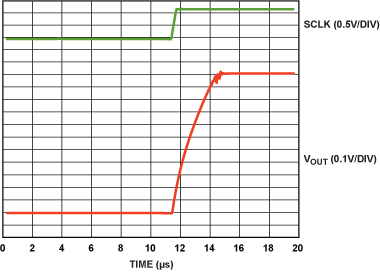Figure 7. Multiplying DAC settling time.Figure 8. AD5541A settling time.

Noise Spectral Density: Table 1 compares noise spectral density of the AD5541A and the multiplying DAC. The AD5541A exhibits slightly better performance at 10 kHz and far better performance at 1 kHz.

Table 1. Noise Spectral Density of AD5541A vs. Multiplying DAC
 DAC NSD @ 10 kHz (nV/√Hz) NSD @ 1 kHz (nV/√Hz) AD5541A 12 12 MDAC 30 140

Integral Nonlinearity: Integral nonlinearity measures the maximum deviation between the ideal output of a DAC and the actual output after gain and offset errors have been removed. The switches used in series with the R-2R network can affect the INL. Multiplying DACs generally employ NMOS switches. When used in voltage-switching mode, the source of the NMOS switch is connected to the reference voltage, the drain is connected to the ladder, and the gate is driven by the internal logic (Figure 9).Figure 9. Multiplying DAC switch.

For current to flow in an NMOS device, VGS must be greater than the threshold voltage, VT. In voltage-switching mode, VGS = VLOGIC – VIN must be greater than VT = 0.7 V.

The R-2R ladder of a multiplying DAC is designed to divide the current evenly through each of the legs. This requires the overall resistance to ground, seen from the top of each leg, to be exactly the same. This can be accomplished by scaling the switches, where the size of each switch is proportional to its on resistance. If the resistance in one leg changes, the current flowing through that leg will change, causing a linearity error. VIN cannot be so large as to shut off the switch, but it must be large enough to keep the switch resistance low, as changes in VIN affect VGS and, therefore, cause a nonlinear change in on resistance as shown by:

This change in on resistance will unbalance the currents and degrade the linearity. Thus, the supply voltage on the multiplying DAC cannot be reduced too much. Conversely, the reference voltage should be no more than 1 V above AGND to maintain linearity. With a 5-V supply, the linearity starts to degrade when moving from a 1.25-V reference to a 2.5-V reference, as shown in Figure 10 and Figure 11. The linearity falls apart altogether when the supply voltage is decreased to 3 V, as shown in Figure 12.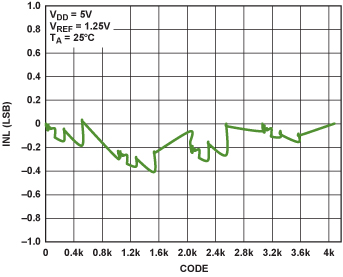Figure 10. INL of IOUT multiplying DAC in reverse mode, VDD = 5 V, VREF = 1.25 V.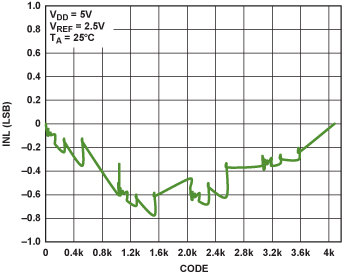Figure 11. INL of IOUT multiplying DAC in reverse mode, VDD = 5 V, VREF = 2.5 V.Figure 12. INL of multiplying DAC in reverse mode, VDD = 3 V, VREF = 2.5 V.

To minimize this effect, the AD5541A uses complementary NMOS/PMOS switches, as shown in Figure 13. Now, the total on resistance of the switch comes from the parallel contribution of the NMOS and PMOS switches. As previously shown, the gate voltage of the NMOS switch is controlled by internal logic. An internally generated voltage, VGN, sets the ideal gate voltage to balance the on resistance of the NMOS with that of the PMOS. The switches are sized to scale with code so the on resistance will scale with code. Thus, the currents will scale, and accuracy will be maintained. As the reference input sees an impedance that varies with code, it should be driven from a low impedance source.Figure 13. Complementary NMOS/PMOS switches.

Figure 14 and Figure 15 show the INL performance of the AD5541A with 5-V and 2.5-V references.Figure 14. INL of AD5541A, VDD = 5.5 V, VREF = 5 V.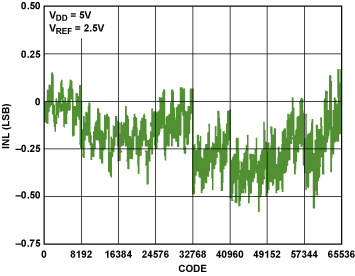Figure 15. INL of AD5541A, VDD = 5.5 V, VREF = 2.5 V.

Figure 16 and Figure 17 show that the linearity changes very little over a wide range of reference and supply voltages. The DNL behavior is similar to that of the INL. The AD5541A linearity is specified over temperature and supply voltage; and the reference voltage can go from 2.5 V to the supply voltage.Figure 16. AD5541A INL vs. supply voltage.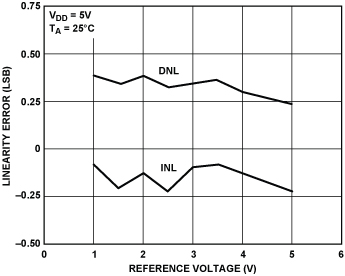Figure 17. AD5541A INL vs. reference voltage.

### More About the AD5541A

The AD5541A serial-input, single-supply, voltage-output nanoDAC+ digital-to-analog converter provides 16-bit resolution with ±0.5-LSB typical integral- and differential nonlinearity. It is well suited to applications that use multiplying DACs in voltage-switching mode. It performs well over both temperature and supply voltage, achieves excellent linearity, and can be used in 3-V to 5-V systems where precision dc performance and quick settling are required. Using an external reference voltage that can range from 2 V to the supply voltage, the unbuffered voltage output can drive a 60-kΩ load from 0 V to VREF. Featuring 1-µs settling to ½ LSB, 11.8-nV/√Hz noise, and low glitch, the device is well suited for deployment in a wide variety of medical, aerospace, communications, and industrial applications. Its 3-wire, low-power SPI-compatible serial interface can be clocked at up to 50 MHz. Operating on a single 2.7-V to 5.5-V supply, the AD5541A draws only 125 µA. Available in 8-lead and 10-lead LFCSP and 10-lead MSOP packages, it is specified from –40°C to +125°C and priced from \$6.25 in 1000s.

### High Speed Current Output DAC Buffers

Transformers are often considered to be the best option for converting the complementary output of a high-speed current-output DAC to a single-ended voltage output, as transformers do not add noise or consume power. Although transformers operate well with high-frequency signals, they cannot handle the low-frequency signals required for many instrumentation and medical applications. These applications require a low-power, low-distortion, low-noise, high-speed amplifier to convert the complementary current to a single-ended voltage. The three circuits presented here accept the complementary output currents from the DAC and provide a single-ended output voltage. Distortion for the last two is compared with a transformer solution.

Difference Amplifier: The AD8129 and AD8130 differential-to-single-ended amplifiers (Figure 1) are used in the first circuit (Figure 2). They feature extremely high common-mode rejection at high frequency. The AD8129 is stable for gains of 10 or more, whereas the AD8130 is stable with unity gain. Their user-adjustable gain can be set by the ratio of two resistors, RF and RG. The AD8129 and AD8130 have very high input impedance on Pin 1 and Pin 8, regardless of the gain setting. A reference voltage (VREF, Pin 4) can be used to set a bias voltage that is multiplied by the same gain as the differential input voltage.Figure 1. AD8129/AD8130 difference amplifiers.Figure 2. DAC buffer using AD8129/AD8130.

Equation 1 and Equation 2 show the relation between the output voltage of the amplifier and the complementary output current of the DAC. The termination resistors, RT, perform a current-to-voltage conversion; the ratio of RF and RG determines the gain. VREF is set to 0 in Equation 2.(1)(2)

In Figure 2, this circuit is applied with a quad high-speed, low-power, 14-bit DAC, where the complementary current-output stage increases the speed and reduces the distortion of low-power DACs.

Figure 3 shows the spurious-free dynamic range (SFDR) of the circuit, as a function of frequency, using the DAC and the AD8129, with RF = 2 kΩ, RG = 221 Ω, RT = 100 Ω, and VO = 8 V p-p, at two values of the supply voltage. Here, the AD8129 was chosen because it provides a large output signal, is stable for G = 10, and has a high gain-bandwidth product compared to the AD8130. The SFDR is generally better than 55 dB for both cases, to beyond 10 MHz, with approximately >3-dB improvement at the lower supply voltage.Figure 3. Distortion of the DAC and AD8129 with VO = 8 V p-p.

Op Amp at Unity Gain: The second circuit (Figure 4) uses a high-speed amplifier with two RT resistors. The amplifier simply transforms the complementary currents, I1 and I2, into a single-ended output voltage, VO, through RT. This simple circuit does not allow signal amplification using the amplifier as a gain block.Figure 4. Simple differential-to-single-ended converter using an op amp.

Equation 3 shows the relationship between VO and the DAC output current. Distortion data was measured with 5-pF capacitors in parallel with RT.(3)

To demonstrate the performance of this circuit, the DAC was paired with the ADA4857 and ADA4817 op amps, with RT = 125 Ω (and CT = CF = 5 pF in parallel with RT for stability and low-pass filtering). The single ADA4857-1 and dual ADA4857-2 are unity-gain stable, high-speed, voltage-feedback amplifiers with low distortion, low noise, and high slew rate. An ideal solution for a variety of applications, including ultrasound, ATE, active filters, and ADC drivers, it features 850-MHz bandwidth, 2800-V/μs slew rate, and 10-ns settling time to 0.1%—all while operating on 5 mA of quiescent current. With a wide supply voltage range (5 V to 10 V), the ADA4857-1 and ADA4857-2 are ideal candidates for systems that require wide dynamic range, precision, high speed, and low power.

The single ADA4817-1 and dual ADA4817-2 FastFET amplifiers are unity-gain stable, ultrahigh-speed, voltage-feedback op amps with FET inputs. Developed on ADI’s proprietary eXtra Fast Complementary Bipolar (XFCB) process, they achieve ultralow noise (4 nV/√Hz and 2.5 fA/√Hz) and very high input impedance. With 1.3-pF input capacitance, 2-mV maximum offset voltage, low power dissipation (19 mA), and wide −3-dB bandwidth (1050 MHz), they are ideal for data acquisition front ends, photodiode preamps, and other wideband transimpedance applications. With a 5-V to 10-V supply voltage range and the ability to operate on either single or dual supplies, they are designed to work in a variety of applications, including active filtering, ADC driving, and DAC buffering.

Figure 5 compares the distortion vs. frequency of this circuit at  VO = 500 mV p-p with a circuit using a transformer. The transformer has less distortion than the amplifier, which has decreasing gain, at high frequencies, but its distortion becomes increasingly worse at low frequencies. Here, SFDRs of nearly 90 dB are achievable over a limited range, with better than 70 dB to 10 MHz.Figure 5. Distortion of the DAC, ADA4857, and ADA4817 with VO = 500 mV p-p, RL = 1 kΩ.

Op Amp with Gain: The third circuit (Figure 6) also uses the same high-speed op amps but includes a resistive network that distances the amplifier from the DAC, allows for gain setting, and has the flexibility to adjust the output bias voltage using either of the two reference voltages, VREF1 and VREF2.Figure 6. Differential-to-single-ended with gain and bias capability.

Equation 4 defines the relationship between the DAC output current and the amplifier output voltage for VREF1 = VREF1 = 0. To match the input impedance of the amplifier network looking out of the DAC, the two termination resistors, RT1 and RT2, must be set individually, taking into consideration the characteristics of the amplifier.(4)

Figure 7 compares the distortion of the amplifiers in this configuration with that of the transformer circuit. RT1 = 143 Ω, RT2 = 200 Ω, RF = RG = 499 Ω, CF = 5 pF—for stability and high frequency filtering—and RL = 1 kΩ. Here, the performance of the ADA4817 is comparable to that of the transformer at high frequency, maintaining better than −70 dBc SFDR up to 70 MHz. Both op amps maintain excellent low-frequency fidelity compared to the transformer.Figure 7. Distortion of the DAC, ADA4817, and ADA4857 with VO = 500 mV p-p.

This article showed some of the advantages of using low-distortion, low-noise, high-speed amplifiers as DAC buffers comparing their performance with that of transformers. It also compares three types of application circuits using two different amplifier architectures, while giving examples of measured data with a DAC and the AD8129, ADA4857-1/ADA4857-2, and ADA4817-1/ADA4817-2 amplifiers. The data show that the amplifier outperforms the transformer at frequencies less than 1 MHz and can closely match its performance up to 80 MHz. Amplifier selection is important when considering trade-offs in terms of power dissipation and distortion.